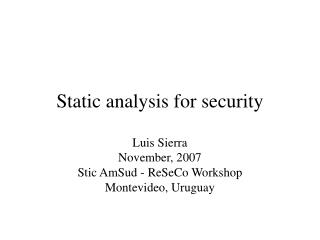DownloadDownload PresentationStatic analysis for security

# Static analysis for security

Télécharger la présentation## Static analysis for security

- - - - - - - - - - - - - - - - - - - - - - - - - - - E N D - - - - - - - - - - - - - - - - - - - - - - - - - - -
##### Presentation Transcript

1. Static analysis for security Luis Sierra November, 2007 Stic AmSud - ReSeCo Workshop Montevideo, Uruguay

2. Plan • Some motivation • Static analysis • PySTA: Python Static Analyzer • Permission usage analysis • Conclusions and further work

3. Motivation • To understand and have experience of the permissions model of Besson, Dufay, and Jensen • Looking for a quick prototype • Moreover, I did not know Python

4. [x := 2]1; [y := 4]2; [x := 1]3; [read (z)]4; if [z > x]5 then [z := y]6 else [z := x]7 Static analysis: example • Take a program S • Take a property P • Check if P holds in every possible execution of S • Checking at compile time • Approximate answers Every assignment is useful

5. Pysta • 1 : set([]) • 2 : set(['y']) • 3 : ... [x := 2]1; [y := 4]2; [x := 1]3; [read (z)]4; if [z > x]5 then [z := y]6 else [z := x]7 Static analysis: example • Check if P holds in every possible execution of S • Checking at compile time Every assignment is useful

6. [x := 2]1; [y := 4]2; [x := 1]3; [read (z)]4; if [z > x]5 then Om; [z := y]6 else [z := x]7 Static analysis: example • Take a program S • Take a property P • If Om does not terminate, we should delete assignment 2. Our analysis solves the halting problem !!! • Checking at compile time • Approximate answers Every assignment is useful

7. The analyzer navigates in the control flow graph, collecting relevant information. This process must terminate

8. Working list • The analyzer navigates in the CFG with an iterator • We exploit Python flexibility defining • an implementation with sets • and an implementation with lists

9. Working list class workingList (object): def iter (self): pass def add (self, c): pass def MFP (a): W = WLmap [WLoption] (a.flow) for (l1, l2) in W.iter (): ... W.add ([(s,t) for (s,t) in ...]) ...

10. Fix-point computation def MFP (a): W = WLmap [WLoption] (a.flow) for (l1, l2) in W.iter (): fl = a.transfer (l1) if (not a.latt.leq (fl, a.a [l2])): a.a [l2] = a.latt.join (a.a [l2], fl) W.add ([(s,t) for (s,t) in a.flow if s==l2]) a.dump ()

11. A static analysis • The analysis is declared in the main program def analyze (file, analysisType): s = open (file + '.xml').read() p = parseString (s).documentElement a = analysisType (p) MFP (a)

12. Some implementations • As well as with working lists, we implemented several static analysis • Live variables • Constraint propagation • Available expressions • Permission usage

13. A class for analysis class MF (object): def __init__ (self, pgm, an): ... def defLattice (self): pass def defextremalValue (self): pass def transfer (self, l, s): pass def initialAnalysis (self): self.a = {} for l in self.Lab: self.a [l] = self.extremalValue \ if l in self.extremalLabel\ else self.latt.bottom () ...

14. Live Variables Analysis class Analysis (MF): def __init__ (self, pgm): MF.__init__ (self, pgm, 'BW') def defLattice (self): self.latt = SetVarLat () def defextremalValue (self): self.extremalValue = SetVar ([]) def transfer (self, l): ... def kill (self, l): return SetVar (eval (getKill (self.Blocks[l]))) def gen (self, l): return SetVar (eval (getGen (self.Blocks[l])))

15. Lattices • The information collected in a static analysis is good enough to provide • an operation of least upper bound (latt.join) • a comparison (latt.leq) • We are not interested in proving that a structure is a lattice, but in implementing quickly the relevant operations

16. A lattice class lattice (object): def U (self): """Support set of the lattice. Meaningful if the support set is finite.""" pass def join (self, a, b): """Join operation: returns a new object with value in the lattice.""" pass def leq (self, a, b): """Less or equal relation: returns True or False.""" pass def bottom (self): """Bottom: returns a new object """ pass

17. A library of lattices class semilattice (object): ... ## a cartesian product using tuples class cartesianProduct (lattice): ... ## a function space class functionspace (lattice): ... ## a function using a dictionary class genFunction (object): ... class newbottom (lattice): ... class powerset (lattice): ... class dual (lattice):

18. 1 0  Multiplicities and permissions

19. Permissions lattice

20. Permissions analysis class Analysis (MF): ... def defLattice (self): self.latt = Perm (self.Resources, self.Action, self.ResType) def defextremalValue (self): self.extremalValue = self.latt.bottom () def transfer (self, l): ... class Perm (functionspace): ...

21. Permissions analysis class Res (powerset): ... class Act (powerset): ... class PermRT (newbottom): ... class Mult (lattice): ... class PermMult (cartesianProduct): ... class RTfunc (oneFunction): ... class Perm (functionspace): ...

22. A program grant (http ('*'), read, inf) grant (https ('site'), read, 1) grant (file ('walletId'), read, 1) while ...: while ...: consume (http ('site'), read) if ...: consume (http ('*'), read) else: break consume (file ('walletId'), read) if ...: consume (http ('site'), read) else: grant (file ('walletVisa'), read, 1) consume (file ('walletVisa'), read) consume (https ('site'), read)

23. PySTA .pgm AE, LV, PU, CP pgmtoxml .xml AE, LV, PU, CP analyze dump